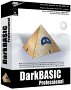# Physics - Forward Kinematics (FK)

Forward Kinematics applies to a chain of jointed objects. Given the angles of the joints, and the distances between them, we want to find the the position of the end point.## Data Structure (using matrix)

If we have the following transforms:

• [Ta]=matrix to transform the joint at the end of link a in local coodinates of link a.
• [Tb]=matrix to transform the joint at the end of link b in local coodinates of link b.
• [Tc]=matrix to transform the calculated end point in local coodinates of link c.

Then the position of the calculated end point in world coordinates is given by multiplying the matrices together:

[Ta][Tb][Tc]

## Data Structure (not using matrix)

We can store the relative positions and orientations of the links in a scenegraph.We can combine translation and rotation without using matrices as described on this page.

• Rtotal = Ra Rb
• Ctotal = Cb
• Ttotal = Ra (Cb + Tb - Ca) + Ca + Tb - Cb

Therefore the parameters for all 3 links gives:

• Rtotal = Ra Rb Rc
• Ctotal = Cc
• Ttotal = Ra (Cb + (Rb (Cc + Tc - Cb) + Cb + Tc - Cc) - Ca) + Ca + (Rb (Cc + Tc - Cb) + Cb + Tc - Cc) - Cb

For a more detailed suggestion of how to store this information see these pages.

So what is the code or algorithm for calculating the endpoint in an n-link chain:

• Rtotal = R1 R2 ... Rn
• Ctotal = Cn
• Ttotal = ???

Can you help me here?

Where I can, I have put links to Amazon for books that are relevant to the subject, click on the appropriate country flag to get more details of the book or to buy it from them.

Commercial Software Shop

Where I can, I have put links to Amazon for commercial software, not directly related to the software project, but related to the subject being discussed, click on the appropriate country flag to get more details of the software or to buy it from them.Dark Basic Professional Edition - It is better to get this professional editionThis is a version of basic designed for building games, for example to rotate a cube you might do the following: make object cube 1,100 for x=1 to 360 rotate object 1,x,x,0 next x

This site may have errors. Don't use for critical systems.